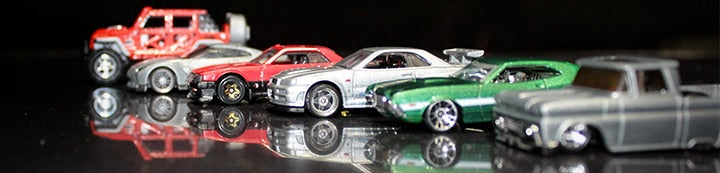1 - 1 of 1 Posts

#### vn1500

·
##### Registered
Joined
·
379 Posts
Discussion Starter
1.9 scale

1 . Jeff (nitrojeff) = -5
2 . Tom (vn1500) = 6
3 . Tony (tklatti) = 16
4 . Nat (nataliemorgan) = 30
5 . Todd (rockcrusher) = 33
6 . Zack (rockcrusherjr) = 35
7 . Dustin (dlcrawler) = 42
8 . Jeff (jeep94xj) = 55
9 . Matt (sloppy) = 64
10 . Stu (oldbustedhotness) = 71

2.2 scale

1 . Tom (vn1500) = 29
2 . Jeff (nitrojeff) = 36
3 . Harley (chuckatruck) = 65
4 . Ed (ed) = 85
5 . Matt (sloppy) = 97
6 . Dustin (dlcrawler) = 168
7 . Nat (nataliemorgan) = 34/dns
8 . Stu (oldbustedhotness) = 42/dns
9 . Tony (tklatti) = 88/dns
10 . Shawn = 101/dns

2.2 sportsman

1 . Tom (vn1500) = 13
2 . Harley (chuckatruck) = 26
3 . Jeremy (bullfrog) = 31
4 . Jeff (nitrojeff) = 42
5 . Ed (ed) = 48
6 . Matt (sloppy) = 58
7 . Jeff (mustangboy_347) = 60
8 . Joe (gijoe) = 64
9 . Nat (nataliemorgan) = 73

2.2 Comp

1 . Jeff (nitrojeff) = 24
2 . Tom (vn1500) = 28
3 . Ed (ed) = 32
4 . Jeff (mustangboy_347) = 63
5 . Jeremy (bullfrog) = 68
6 . Kyle (superduper) = 70
7 . Travis (Tbredon) = 75

Awesome day of crawlin everybody , it's too bad some people had to beat it . Courses were tough , couple of the gates that seemed impossible weren't and a couple of pulls that shoulda been a walk in the park turned out to be real MF ers to get through ... See everybody in 2 weeks ...
__________________

1.9 Scale TLT
1.9 Comp MRC
2.2 Scale W/K
2.2 Sportsman AX-10
2.2 Comp Berg

N.O.S.T.R.
O.C.D.

1 - 1 of 1 Posts